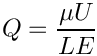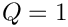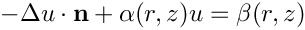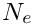oomph::Ellipse Class Reference

//////////////////////////////////////////////////////////////////// More...

#include <geom_objects.h>Inheritance diagram for oomph::Ellipse:

## Public Member Functions

Ellipse (const Vector< Data * > &geom_data_pt)
Constructor: 1 Lagrangian coordinate, 2 Eulerian coords. Pass half axes as Data: More...

Ellipse (const double &A, const double &B)
Constructor: 1 Lagrangian coordinate, 2 Eulerian coords. Pass half axes A and B; both pinned. More...

Ellipse (const Ellipse &dummy)=delete
Broken copy constructor. More...

void operator= (const Ellipse &)=delete
Broken assignment operator. More...

~Ellipse ()
Destructor: Clean up if necessary. More...

void set_A_ellips (const double &a)
Set horizontal half axis. More...

void set_B_ellips (const double &b)
Set vertical half axis. More...

double a_ellips ()
Access function for horizontal half axis. More...

double b_ellips ()
Access function for vertical half axis. More...

void position (const Vector< double > &zeta, Vector< double > &r) const
Position Vector at Lagrangian coordinate zeta. More...

void position (const unsigned &t, const Vector< double > &zeta, Vector< double > &r) const
Parametrised position on object: r(zeta). Evaluated at previous timestep. t=0: current time; t>0: previous timestep. More...

void dposition (const Vector< double > &zeta, DenseMatrix< double > &drdzeta) const
Derivative of position Vector w.r.t. to coordinates:= drdzeta(alpha,i). More...

void d2position (const Vector< double > &zeta, RankThreeTensor< double > &ddrdzeta) const
2nd derivative of position Vector w.r.t. to coordinates:= ddrdzeta(alpha,beta,i). Evaluated at current time. More...

void d2position (const Vector< double > &zeta, Vector< double > &r, DenseMatrix< double > &drdzeta, RankThreeTensor< double > &ddrdzeta) const
Position Vector and 1st and 2nd derivs to coordinates:= drdzeta(alpha,i).= ddrdzeta(alpha,beta,i). Evaluated at current time. More...

unsigned ngeom_data () const
How many items of Data does the shape of the object depend on? More...

Datageom_data_pt (const unsigned &j)
Return pointer to the j-th Data item that the object's shape depends on. More...Public Member Functions inherited from oomph::GeomObject
GeomObject ()
Default constructor. More...

GeomObject (const unsigned &ndim)
Constructor: Pass dimension of geometric object (# of Eulerian coords = # of Lagrangian coords; no time history available/needed) More...

GeomObject (const unsigned &nlagrangian, const unsigned &ndim)
Constructor: pass # of Eulerian and Lagrangian coordinates. No time history available/needed. More...

GeomObject (const unsigned &nlagrangian, const unsigned &ndim, TimeStepper *time_stepper_pt)
Constructor: pass # of Eulerian and Lagrangian coordinates and pointer to time-stepper which is used to handle the position at previous timesteps and allows the evaluation of veloc/acceleration etc. in cases where the GeomData varies with time. More...

GeomObject (const GeomObject &dummy)=delete
Broken copy constructor. More...

void operator= (const GeomObject &)=delete
Broken assignment operator. More...

virtual ~GeomObject ()
(Empty) destructor More...

unsigned nlagrangian () const
Access function to # of Lagrangian coordinates. More...

unsigned ndim () const
Access function to # of Eulerian coordinates. More...

void set_nlagrangian_and_ndim (const unsigned &n_lagrangian, const unsigned &n_dim)
Set # of Lagrangian and Eulerian coordinates. More...

TimeStepper *& time_stepper_pt ()
Access function for pointer to time stepper: Null if object is not time-dependent. More...

TimeSteppertime_stepper_pt () const
Access function for pointer to time stepper: Null if object is not time-dependent. Const version. More...

virtual void position (const double &t, const Vector< double > &zeta, Vector< double > &r) const
Parametrised position on object: r(zeta). Evaluated at the continuous time value, t. More...

virtual void dposition_dt (const Vector< double > &zeta, const unsigned &j, Vector< double > &drdt)
j-th time-derivative on object at current time:. More...

virtual void locate_zeta (const Vector< double > &zeta, GeomObject *&sub_geom_object_pt, Vector< double > &s, const bool &use_coordinate_as_initial_guess=false)
A geometric object may be composed of may sub-objects (e.g. a finite-element representation of a boundary). In order to implement sparse update functions, it is necessary to know the sub-object and local coordinate within that sub-object at a given intrinsic coordinate, zeta. Note that only one sub-object can "cover" any given intrinsic position. If the position is at an "interface" between sub-objects, either one can be returned. The default implementation merely returns, the pointer to the "entire" GeomObject and the coordinate, zeta The optional boolean flag only applies if a Newton method is used to find the value of zeta, and if true the value of the coordinate s is used as the initial guess for the method. If the flag is false (the default) a value of s=0 is used as the initial guess. More...

virtual void interpolated_zeta (const Vector< double > &s, Vector< double > &zeta) const
A geometric object may be composed of many sub-objects each with their own local coordinate. This function returns the "global" intrinsic coordinate zeta (within the compound object), at a given local coordinate s (i.e. the intrinsic coordinate of the sub-GeomObject. In simple (non-compound) GeomObjects, the local intrinsic coordinate is the global intrinsic coordinate and so the function merely returns s. To make it less likely that the default implementation is called in error (because it is not overloaded in a derived GeomObject where the default is not appropriate, we do at least check that s and zeta have the same size if called in PARANOID mode. More...

## Private Attributes

Vector< Data * > Geom_data_pt
Vector of pointers to Data items that affects the object's shape. More...

bool Must_clean_up
Do I need to clean up? More...Protected Attributes inherited from oomph::GeomObject
unsigned NLagrangian
Number of Lagrangian (intrinsic) coordinates. More...

unsigned Ndim
Number of Eulerian coordinates. More...

TimeStepperGeom_object_time_stepper_pt

## Detailed Description

////////////////////////////////////////////////////////////////////

//////////////////////////////////////////////////////////////////// Steady ellipse with half axes A and B as geometric object:Definition at line 643 of file geom_objects.h.

## ◆ Ellipse() [1/3]

 oomph::Ellipse::Ellipse ( const Vector< Data * > & geom_data_pt )
inline

Constructor: 1 Lagrangian coordinate, 2 Eulerian coords. Pass half axes as Data:

Geom_data_pt->value(0) = A
Geom_data_pt->value(1) = B
Vector< Data * > Geom_data_pt
Vector of pointers to Data items that affects the object's shape.
Definition: geom_objects.h:853

Definition at line 652 of file geom_objects.h.

References geom_data_pt(), Geom_data_pt, Must_clean_up, and oomph::Data::nvalue().

## ◆ Ellipse() [2/3]

 oomph::Ellipse::Ellipse ( const double & A, const double & B )
inline

Constructor: 1 Lagrangian coordinate, 2 Eulerian coords. Pass half axes A and B; both pinned.

Definition at line 682 of file geom_objects.h.

References oomph::OcTreeNames::B, Geom_data_pt, and Must_clean_up.

## ◆ Ellipse() [3/3]

 oomph::Ellipse::Ellipse ( const Ellipse & dummy )
delete

Broken copy constructor.

## ◆ ~Ellipse()

 oomph::Ellipse::~Ellipse ( )
inline

Destructor: Clean up if necessary.

Definition at line 709 of file geom_objects.h.

References Geom_data_pt, and Must_clean_up.

## ◆ a_ellips()

 double oomph::Ellipse::a_ellips ( )
inline

Access function for horizontal half axis.

Definition at line 732 of file geom_objects.h.

References Geom_data_pt.

## ◆ b_ellips()

 double oomph::Ellipse::b_ellips ( )
inline

Access function for vertical half axis.

Definition at line 738 of file geom_objects.h.

References Geom_data_pt.

## ◆ d2position() [1/2]

 void oomph::Ellipse::d2position ( const Vector< double > & zeta, RankThreeTensor< double > & ddrdzeta ) const
inlinevirtual

2nd derivative of position Vector w.r.t. to coordinates:= ddrdzeta(alpha,beta,i). Evaluated at current time.

Reimplemented from oomph::GeomObject.

Definition at line 804 of file geom_objects.h.

References Geom_data_pt.

## ◆ d2position() [2/2]

 void oomph::Ellipse::d2position ( const Vector< double > & zeta, Vector< double > & r, DenseMatrix< double > & drdzeta, RankThreeTensor< double > & ddrdzeta ) const
inlinevirtual

Position Vector and 1st and 2nd derivs to coordinates:= drdzeta(alpha,i).= ddrdzeta(alpha,beta,i). Evaluated at current time.

Reimplemented from oomph::GeomObject.

Definition at line 817 of file geom_objects.h.

References Geom_data_pt.

## ◆ dposition()

 void oomph::Ellipse::dposition ( const Vector< double > & zeta, DenseMatrix< double > & drdzeta ) const
inlinevirtual

Derivative of position Vector w.r.t. to coordinates:= drdzeta(alpha,i).

Reimplemented from oomph::GeomObject.

Definition at line 791 of file geom_objects.h.

References Geom_data_pt.

## ◆ geom_data_pt()

 Data * oomph::Ellipse::geom_data_pt ( const unsigned & j )
inlinevirtual

Return pointer to the j-th Data item that the object's shape depends on.

Reimplemented from oomph::GeomObject.

Definition at line 846 of file geom_objects.h.

References Geom_data_pt.

Referenced by Ellipse().

## ◆ ngeom_data()

 unsigned oomph::Ellipse::ngeom_data ( ) const
inlinevirtual

How many items of Data does the shape of the object depend on?

Reimplemented from oomph::GeomObject.

Definition at line 839 of file geom_objects.h.

References Geom_data_pt.

## ◆ operator=()

 void oomph::Ellipse::operator= ( const Ellipse & )
delete

Broken assignment operator.

## ◆ position() [1/2]

 void oomph::Ellipse::position ( const unsigned & t, const Vector< double > & zeta, Vector< double > & r ) const
inlinevirtual

Parametrised position on object: r(zeta). Evaluated at previous timestep. t=0: current time; t>0: previous timestep.

Reimplemented from oomph::GeomObject.

Definition at line 756 of file geom_objects.h.

References Geom_data_pt, Must_clean_up, position(), t, and oomph::GeomObject::time_stepper_pt().

## ◆ position() [2/2]

 void oomph::Ellipse::position ( const Vector< double > & zeta, Vector< double > & r ) const
inlinevirtual

Position Vector at Lagrangian coordinate zeta.

Implements oomph::GeomObject.

Definition at line 745 of file geom_objects.h.

References Geom_data_pt.

Referenced by position(), and oomph::TwoDAnnularMesh< ELEMENT >::wrap_into_annular_shape().

## ◆ set_A_ellips()

 void oomph::Ellipse::set_A_ellips ( const double & a )
inline

Set horizontal half axis.

Definition at line 720 of file geom_objects.h.

References Geom_data_pt.

## ◆ set_B_ellips()

 void oomph::Ellipse::set_B_ellips ( const double & b )
inline

Set vertical half axis.

Definition at line 726 of file geom_objects.h.

References Geom_data_pt.

## ◆ Geom_data_pt

 Vector oomph::Ellipse::Geom_data_pt
private

Vector of pointers to Data items that affects the object's shape.

Definition at line 853 of file geom_objects.h.

## ◆ Must_clean_up

 bool oomph::Ellipse::Must_clean_up
private

Do I need to clean up?

Definition at line 856 of file geom_objects.h.

Referenced by Ellipse(), position(), and ~Ellipse().

The documentation for this class was generated from the following file: